## PyTorch-专知-链路化知识-3、< 快速理解系列 (二): 图文 代码, 让你快速理解 LSTM>

### 3、< 快速理解系列(二): 图文+代码, 让你快速理解LSTM>

LSTM（Long Short-Term Memory）是长短期记忆网络，是一种时间递归神经网络，适合于处理和预测时间序列中间隔和延迟相对较长的重要事件。 LSTM 已经在科技领域有了多种应用。基于 LSTM 的系统可以学习翻译语言、控制机器人、图像分析、文档摘要、语音识别图像识别、手写识别、控制聊天机器人、预测疾病、点击率和股票、合成音乐等等任务。

#### 1、Recurrent Neural Networks

RNN 解决了这个问题。RNN 是包含循环的网络，允许信息的持久化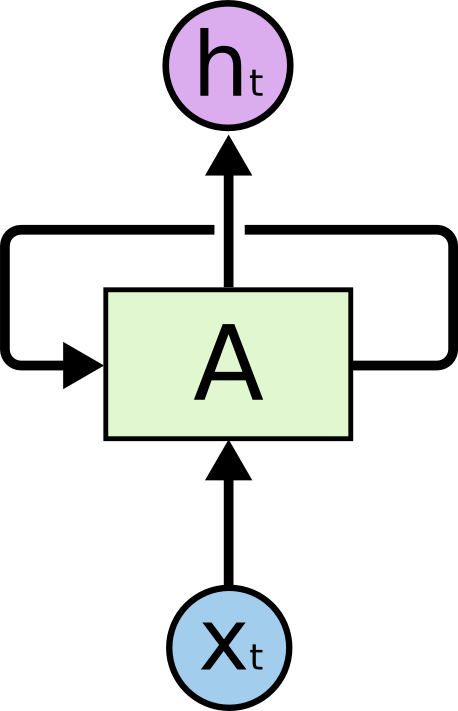An unrolled recurrent neural network

#### 2、长期依赖（Long-Term Dependencies）问题

RNN的出现主要是它们能够把之前的信息联系到现在的任务中去，例如使用过去的视频段来推测对当前段的理解。如果RNN可以做到这个，他们就变得非常有用。但是真的可以么？答案是，还有很多依赖因素。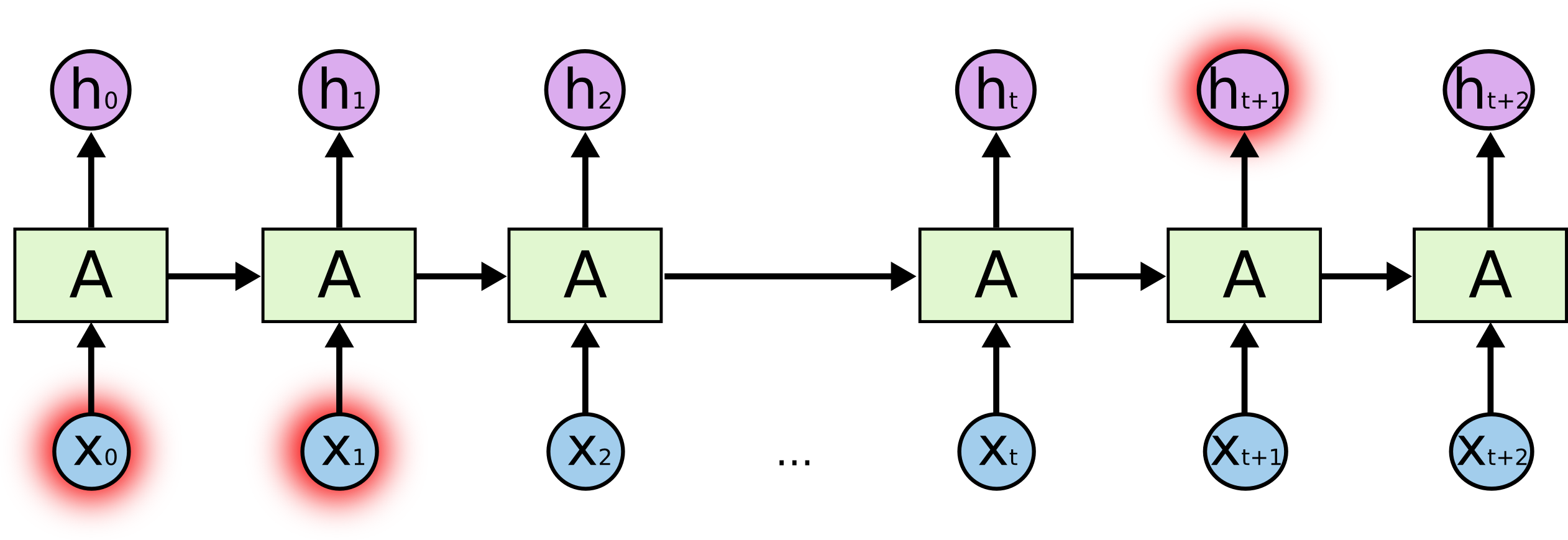#### 3、LSTM

Long Short Term 网络（通常称为“LSTM”）是一种特殊的RNN，能够学习长期的依赖关系。他们由Hochreiter＆Schmidhuber（1997）介绍，并被许多人完善和推广。他们在各种各样的问题上工作得非常好，现在被广泛使用。

LSTM被明确设计为避免长期依赖问题。记住长时间的信息实际上是他们的默认行为，而不是他们难以学习的东西！

The repeating module in a standard RNN contains a single layer

LSTM 同样是这样的结构，但是重复的模块拥有一个不同的结构。不同于 单一神经网络层，这里是有四个，以一种非常特殊的方式进行交互。center>The repeating module in an LSTM contains four interacting layers.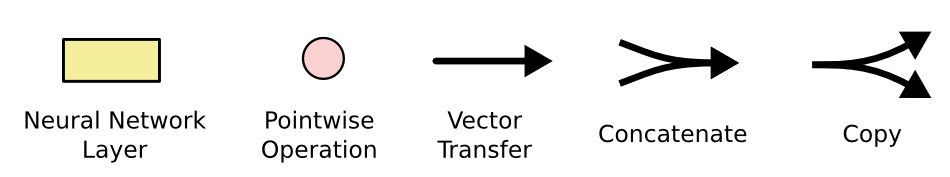#### 4、LSTM 的核心思想

LSTM的关键是细胞状态，水平线穿过图的顶部。

cell状态类似于输送带。它直接在整个链上运行，只有一些少量的线性相互作用。信息在上面流传保持不变会很容易。LSTM具有删除或添加信息到cell状态的能力，这被称为“门”的结构去调节。 门是一种让信息选择式通过的方法。他们包含一个sigmoid神经网络层和一个 pointwise 乘法操作。sigmoid层输出（是一个向量）的每个元素都是一个在 0 和 1 之间的实数，表示让对应信息通过的权重（或者占比）。比如， 0 表示“不让任何信息通过”， 1 表示“让所有信息通过”。

LSTM 拥有三个门，来保护和控制细胞状态。

#### 5、逐步理解 LSTM

##### 1、遗忘门（forget gate）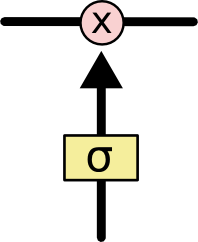##### 2、输入门（input gate）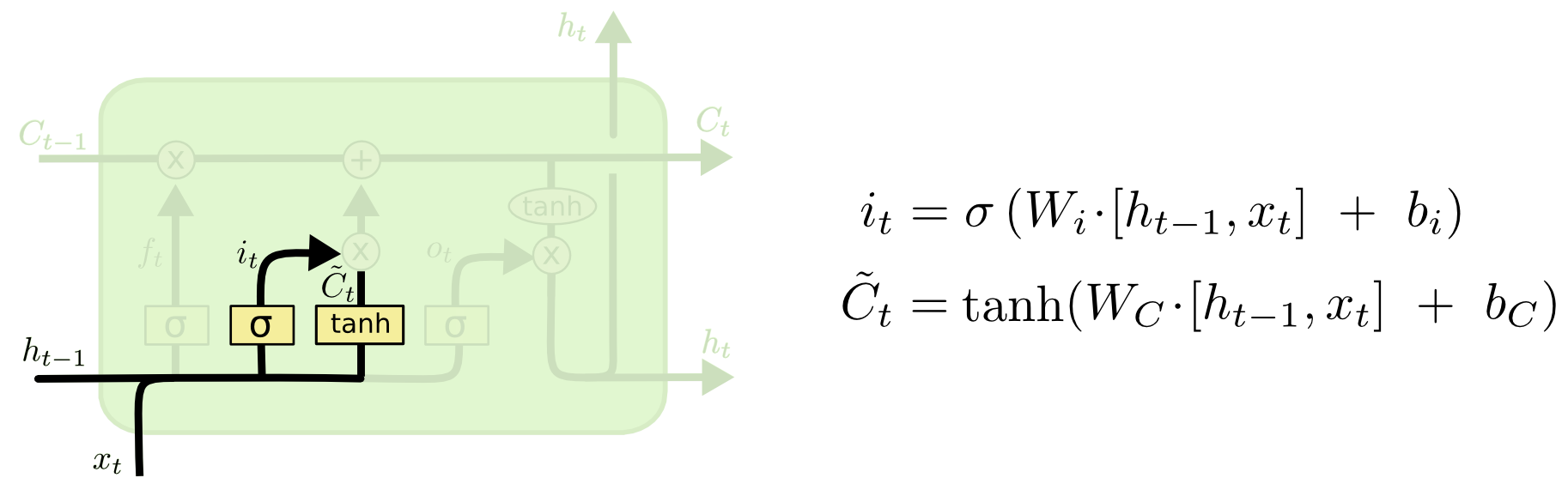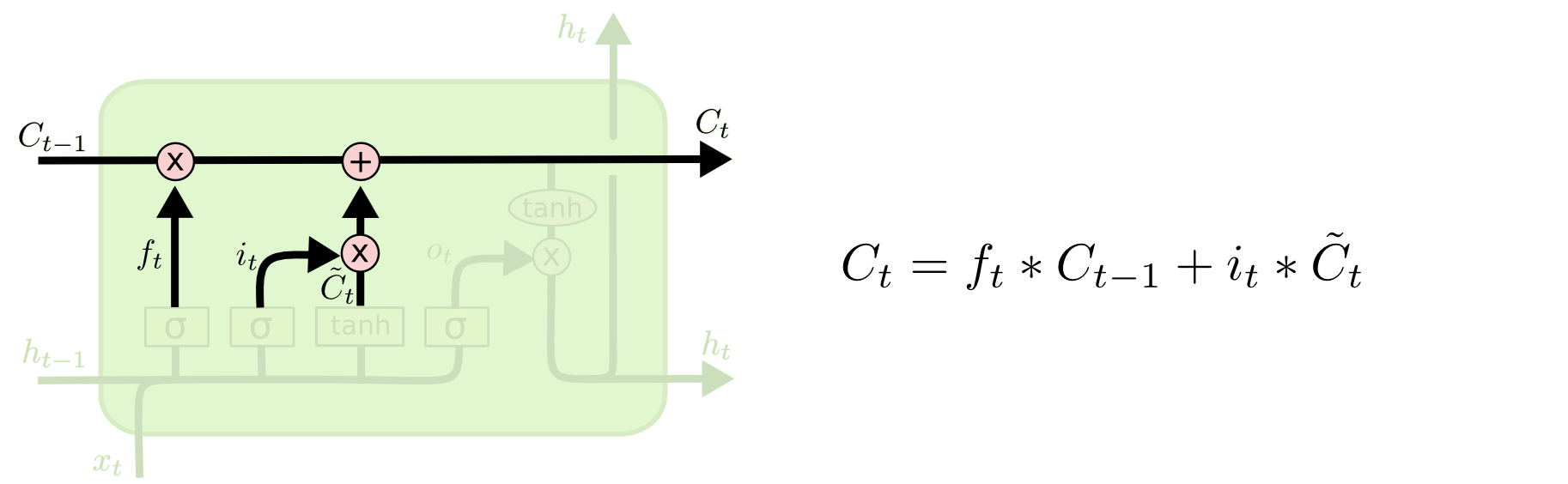#### 3、输出门（output gate）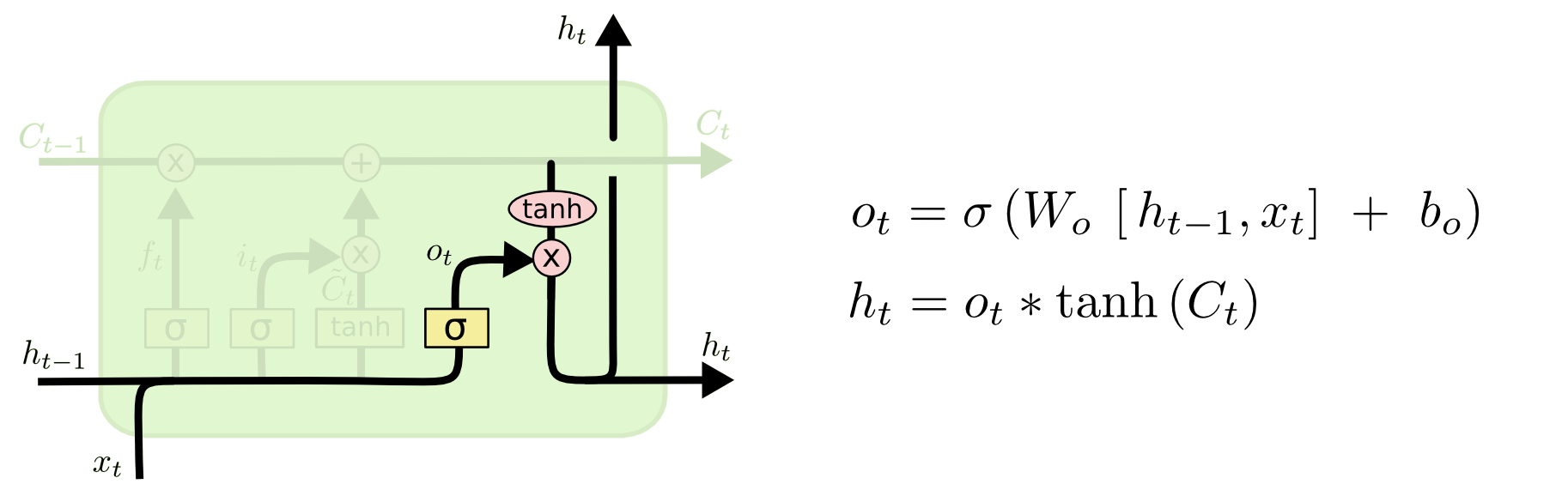#### 6、LSTM 的变体

LSTM的一个稍微更显着的变化是由Cho，et al(2014)介绍的门控循环单元——GRU。 。它将忘记和输入门组合成一个单一的“更新门”，它还合并了cell状态和隐藏状态，并进行了一些其他更改。所得到的模型比标准LSTM模型更简单，并且越来越受欢迎。#### 7、结论

LSTM是我们用RNN获得的重要成功。很自然的想法：还会有更大的突破吗？研究人员的共同观点是：“是的！有一个下一步，那就是Attention！“这个想法是让RNN的每个步骤从一些较大的信息集合中挑选信息。例如，如果您使用RNN创建描述图像的标题，则可能会选择图像的一部分来查看其输出的每个字。事实上，Xu, et al.(2015)做到这一点.如果你想要深入探索Attention，这可能是一个有趣的起点！已经有一些使用 Attention令人兴奋的结果，似乎更多的还值得我们去探索...

Attention不是RNN研究中唯一令人兴奋的线索。例如Kalchbrenner, et al(2015)提出的Grid LSTM似乎很有前途。使用生成模型的RNN，诸如Gregor, et al. (2015)Chung, et al. (2015)Bayer & Osendorfer (2015)提出的模型 也似乎很有趣。最近几年对于RNN来说已经是一个激动人心的时刻了，而后来的研究成果将会更加丰富。

#### 8、PyTorch实现

• 循环神经网络 RNN 和 Bi-RNN
• 实现MNIST的分类

import torch
from torch import nn
from torch.autograd import Variable
import torchvision.datasets as dsets
import torchvision.transforms as transforms
import matplotlib.pyplot as plt

torch.manual_seed(1)
# 超参
num_epochs = 1
batch_size = 64

TIME_STEP = 28        # rnn time step, 即图片的高
INPUT_SIZE = 28       # rnn input size, 即图片的宽
HIDDEN_SIZE = 64
NUM_LAYERS = 2
NUM_CLASSES = 10
BIDIRECTIONAL = True  # 是否开启双向

learning_rate = 0.01

train_data = dsets.MNIST(
root='./mnist/',
train=True,
transform=transforms.ToTensor(),
download=True
)

# 看一下数据
print(train_data.train_data.size())    # (60000, 28, 28)
print(train_data.train_labels.size())  # (60000)
plt.imshow(train_data.train_data.numpy(), cmap='gray')
plt.title('%i' % train_data.train_labels)
plt.show()

train_loader = torch.utils.data.DataLoader(dataset=train_data, batch_size=batch_size, shuffle=True)

test_data = dsets.MNIST(
root='./mnist/',
train=False,
transform=transforms.ToTensor()
)
# test_x shape (-1, 28, 28) value in range(0,1)
test_x = Variable(test_data.test_data, volatile=True).type(torch.FloatTensor) / 255.
test_y = test_data.test_labels
if torch.cuda.is_available():
test_x = test_x.cuda()
test_y = test_y.cuda()

class RNN(nn.Module):
def __init__(self, input_size, hidden_size, num_layers, num_classes, bidirectional):
super(RNN, self).__init__()

self.rnn = nn.LSTM(
input_size=input_size,
hidden_size=hidden_size,     # 隐层单元数
num_layers=num_layers,       # 层数
batch_first=True,  # 第一个维度设为 batch, 即:(batch, time_step, input_size)
bidirectional=bidirectional  # 是否用双向
)

self.out = nn.Linear(hidden_size * 2, num_classes) if bidirectional else nn.Linear(hidden_size, num_classes)

def forward(self, x):
# x: (batch, time_step, input_size)
# r_out: (batch, time_step, output_size)
# h_n: (n_layers, batch, hidden_size)
# h_c: (n_layers, batch, hidden_size)
r_out, (h_n, h_c) = self.rnn(x, None)  # None即隐层状态用0初始化

# 我们只需要最后一步的输出, 即(batch, -1, output_size)
out = self.out(r_out[:, -1, :])
return out
rnn = RNN(
input_size=INPUT_SIZE,
hidden_size=HIDDEN_SIZE,
num_layers=NUM_LAYERS,
num_classes=NUM_CLASSES,
bidirectional=BIDIRECTIONAL
)
print(rnn)

if torch.cuda.is_available():
rnn = rnn.cuda()

optimizer = torch.optim.Adam(rnn.parameters(), lr=learning_rate)
loss_func = nn.CrossEntropyLoss()

for num_epochs in range(num_epochs):
for step, (x, y) in enumerate(train_loader):
b_x = Variable(x.view(-1, 28, 28))  # reshape x to (batch, time_step, input_size)
b_y = Variable(y)
if torch.cuda.is_available():
b_x = b_x.cuda()
b_y = b_y.cuda()

output = rnn(b_x)
loss = loss_func(output, b_y)
optimizer.zero_grad()
loss.backward()
optimizer.step()

if step % 50 == 0:
test_output = rnn(test_x)  # (samples, time_step, input_size)
pred_y = torch.max(test_output, dim=1).data.squeeze()
accuracy = sum(pred_y == test_y) / float(test_y.size(0))
print('num_epochs: ', num_epochs, '| train loss: %.4f' % loss.data, '| test accuracy: %.2f' % accuracy)

# 看一下10个结果
test_output = rnn(test_x[:10].view(-1, 28, 28))
pred_y = torch.max(test_output, 1).data.cpu().numpy().squeeze()
print(pred_y, '预测值')
print(test_y[:10].cpu().numpy().squeeze(), '真实值')Top